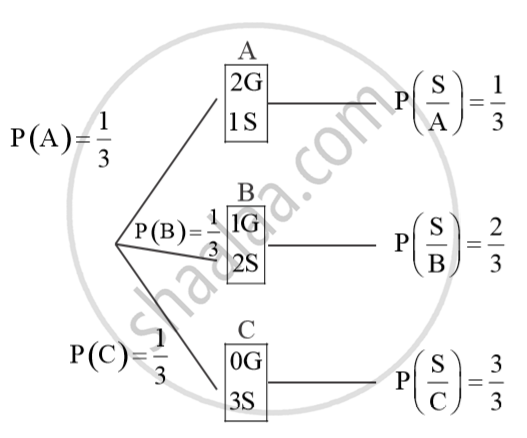# Given Three Identical Boxes A, B and C, Box a Contains 2 Gold and 1 Silver Coin, Box B Contains 1 Gold and 2 Silver Coins and Box C Contains 3 Silver Coins. a Person Choose a Box at Random and - Mathematics

Sum

Given three identical Boxes A, B and C, Box A contains 2 gold and 1 silver coin, Box B contains 1 gold and 2 silver coins and Box C contains 3 silver coins. A person choose a Box at random and takes out a coin. If the coin drawn is of silver, find the probability that it has been drawn from the Box which has the remaining two coins also of silver.

#### SolutionUsing Baye’s theorem
therefore P(C/S) = P(C)xx(P(S/C))/(P(A)xxP(B)xxP(S/B)+P(C)xxP(S/C))
= (1/3 xx 3/3)/(1/3xx1/3+1/3xx2/3 +1/3xx3/3)

= 1/(1/3+2/3+3/3

= 1/(6/3)

1/2

Concept: Introduction of Integrals
Is there an error in this question or solution?